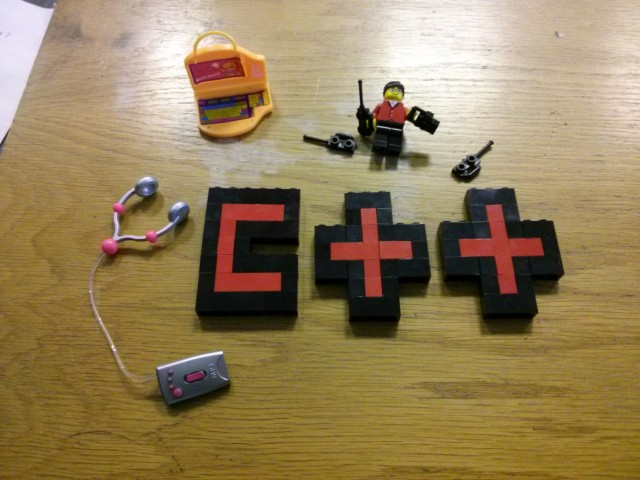# C++: What are the differences between a pointer variable and a reference variable in C++?I know references are syntactic sugar, so code is easier to read and write.

But what are the differences?

1. A pointer can be re-assigned any number of times while a reference can not be re-seated after binding.
2. Pointers can point nowhere (`NULL`), whereas reference always refer to an object.
3. You can’t take the address of a reference like you can with pointers.
4. There’s no “reference arithmetics” (but you can take the address of an object pointed by a reference and do pointer arithmetics on it as in `&obj + 5`).

To clarify a misconception:

The C++ standard is very careful to avoid dictating how a compiler must implement references, but every C++ compiler implements references as pointers. That is, a declaration such as:

``int &ri = i;``

if it’s not optimized away entirely, allocates the same amount of storage as a pointer, and places the address of i into that storage.

So, a pointer and a reference both occupy the same amount of memory.

As a general rule,

• Use references in function parameters and return types to define useful and self-documenting interfaces.
• Use pointers to implement algorithms and data structures.

prakash

1. A pointer can be re-assigned:
``````int x = 5;
int y = 6;
int *p;
p =  &x;
p = &y;
*p = 10;
assert(x == 5);
assert(y == 10);``````

A reference cannot, and must be assigned at initialization:

``````int x = 5;
int y = 6;
int &r = x;``````
2. A pointer has its own memory address and size on the stack (4 bytes on x86), whereas a reference shares the same memory address (with the original variable) but also takes up some space on the stack. Since a reference has the same address as the original variable itself, it is safe to think of a reference as another name for the same variable. Note: What a pointer points to can be on the stack or heap. Ditto a reference. My claim in this statement is not that a pointer must point to the stack. A pointer is just a variable that holds a memory address. This variable is on the stack. Since a reference has its own space on the stack, and since the address is the same as the variable it references. More on stack vs heap. This implies that there is a real address of a reference that the compiler will not tell you.
``````int x = 0;
int &r = x;
int *p = &x;
int *p2 = &r;
assert(p == p2);``````
3. You can have pointers to pointers to pointers offering extra levels of indirection. Whereas references only offer one level of indirection.
``````int x = 0;
int y = 0;
int *p = &x;
int *q = &y;
int **pp = &p;
pp = &q;//*pp = q
**pp = 4;
assert(y == 4);
assert(x == 0);``````
4. Pointer can be assigned NULL directly, whereas reference cannot. If you try hard enough, and you know how, you can make the address of a reference NULL. Likewise, if you try hard enough you can have a reference to a pointer, and then that reference can contain NULL.
``````int *p = NULL;
int &r = NULL; <--- compiling error``````
5. Pointers can iterate over an array, you can use `++` to go to the next item that a pointer is pointing to, and `+ 4` to go to the 5th element. This is no matter what size the object is that the pointer points to.
6. A pointer needs to be dereferenced with `*` to access the memory location it points to, whereas a reference can be used directly. A pointer to a class/struct uses `->` to access it’s members whereas a reference uses a `.`.
7. A pointer is a variable that holds a memory address. Regardless of how a reference is implemented, a reference has the same memory address as the item it references.
8. References cannot be stuffed into an array, whereas pointers can be (Mentioned by user @litb)
9. Const references can be bound to temporaries. Pointers cannot (not without some indirection):
``````const int &x = int(12); //legal C++
int *y = &int(12); //illegal to dereference a temporary.``````

This makes `const&` safer for use in argument lists and so forth.

Brian R. Bondy

Stack Overflow